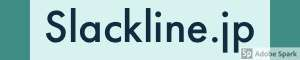# Quick Slackline Tension Calculator for smartphone

この記事は約 3 分で読めます。 投稿から 6年。最終更新から 1年経過。

## Slackline Tension Calculator

You can input quickly with a smartphone.
Of course it is also possible on PC.

Calculate the tension of the slackline from the length/sag/weight.

Calculate Exact(direction) force.
Longline & rodeoline & sagline are also practical.

The weight of the line & backup rope include for super long highline.

↑ここに計算入力フォームが読み込まれるはずですが、エラーにより出ない場合があります。

### Method of calculation

#### EXACT line tension

$$T=\frac{B+\frac{E}{2}\sqrt{S^2 + (\frac{H}{2})^2}} {2S}$$

T :Tension (exact)
B :Body Weight
E :Line Weight (total)
H :Horizontal length
S :Sag

1kgf＝9.81N＝0.00981kN

#### Horizontal line tension

$$T=\frac{H×(B+\frac{E}{2})}{4S}$$

T :Tension (Horizontal)
B:Body Weight
E :Line Weight (total)
H :Horizontal length
S :Sag

1kgf＝9.81N＝0.00981kN

#### Exact line length

$$L = 2\sqrt{(\frac{H}{2})^2+S^2}$$

L :Exact line length
H :Horizontal length
S :Sag

#### Angle of anchor point

$$A = \tan{^{-1}} \frac{S}{\frac{H}{2}}$$

A :Angle of anchor
H :Horizontal length
S :Sag

#### Angle of middle point

$$M = (90- A)×2$$

M:Angle of middle point
A:Angle of anchor

#### Special thanks

Super Slacker Resources

### Weight of the line

SlackDb - Slackline gear
Slackline gear list

## Related tools

スポンサーリンクスラックラインの歩き方